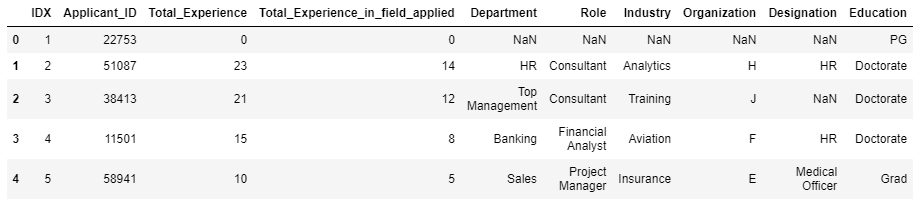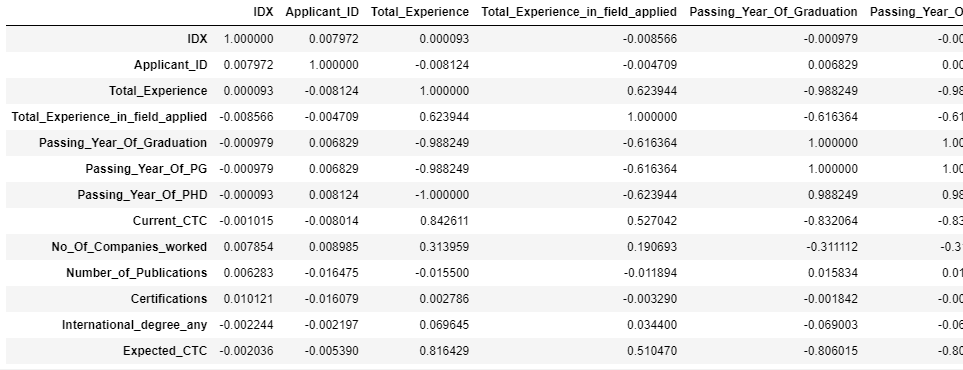top of page
Search

# Important Machine Learning Practice Set | Get Help In Machine LearningQuestion-1

You have been given comprehensive data of expected CTC.

Your task is to analyse this data and figure and also to check if you can build a model .

1. Perform EDA on the data

2. Split the data into train and test (70:30)

3. Build regression models (at least 2 different model)

4. Interpret model results

Question-2

This dataset contains subjects classification. It includes two columns, "Questions" and "Subjects". Perform analysis on the data and figure.

• Language Detection

• Named Entity Recognition

• Data pre processing

Extract Uni-Gram features (retain only 500 columns) by performing pre-processing

• Split the data into train and test (70:30)

• Build classification models (at least 2 different model)

• Interpret model results

Rubric Criteria

1a) Perform EDA on the data This area will be used by the assessor to leave comments related to this criterion.

1b) Split the data into train and test (70:30) This area will be used by the assessor to leave comments related to this criterion.

1c) Build regression models This area will be used by the assessor to leave comments related to this criterion.

1d) Interpret model results This area will be used by the assessor to leave comments related to this criterion.

2a) Language Detection This area will be used by the assessor to leave comments related to this criterion.

2b) Named Entity Recognition This area will be used by the assessor to leave comments related to this criterion.

2c) Data Pre-Processing This area will be used by the assessor to leave comments related to this criterion.

2d) Extract Uni-Gram features This area will be used by the assessor to leave comments related to this criterion.

2e) Split the data into train and test (70:30) This area will be used by the assessor to leave comments related to this criterion.

2f) Build classification models This area will be used by the assessor to leave comments related to this criterion.

2g) Interpret model results This area will be used by the assessor to leave comments related to this criterion.

Implementation

Import all necessary packages

```import pandas as pd # package for high-performance, easy-to-use data structures and data analysis
import numpy as np # fundamental package for scientific computing with Python
import matplotlib
import matplotlib.pyplot as plt # for plotting
import seaborn as sns # for making plots with seaborn
color = sns.color_palette()
import plotly.offline as py
py.init_notebook_mode(connected=True)
from plotly.offline import init_notebook_mode, iplot
init_notebook_mode(connected=True)
import plotly.graph_objs as go
import plotly.offline as offline```

```#reading data

Display Data

```#glimpse of data

output:```#dataset info
d1.info()```

output:...

...

...

```# checking missing data
total = d1.isnull().sum().sort_values(ascending = False)
percent = (d1.isnull().sum()/d1.isnull().count()*100).sort_values(ascending = False)
d1_train_data  = pd.concat([total, percent], axis=1, keys=['Total', 'Percent'])

output:...

...

we have highest nymber of null values in Passing_Year_Of_PHD

PHD_Specialization,University_PHD these 3 columns

```
#let's drp null values
d1=d1.dropna()
d1```

output:```#correlation matrix
d1.corr()```

output:1a) Perform EDA on the data This area will be used by the assessor to leave comments related to this criterion

```#heatmap visualization
plt.figure(figsize = (16,8))
ax = sns.heatmap(d1.corr(), annot=True)```

output:Passing_Year_of_graduation and Passing_Year_of_PG both these columns are highly correlated(1) ,and Expected_CTC and Total_Experience are also highly positively correlated(0.82), Current_CTC and Total_Experience

```# Department of employees
plt.figure(figsize=(10,10))
dept=  d1['Department'].value_counts().reset_index()
ax = sns.barplot("Department","index",data=dept[:20],linewidth=2,edgecolor="k"*10)
plt.xlabel("number of employees")
plt.ylabel("Department")
plt.title("Departments with highest number of employees")
plt.grid(True,alpha=.3)

for i,j in enumerate(dept["Department"][:20]):
ax.text(.7,i,j,weight = "bold")```

output:Marketing and Sales department have highest number of Employees

To get the complete solution of above problem you can get comment in below comment section or send your requirement details at:

realcode4you@gmail.com